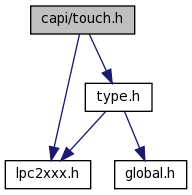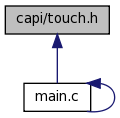# capi/touch.h File Reference

`#include <type.h>`
`#include <lpc2xxx.h>`
Include dependency graph for touch.h:This graph shows which files directly or indirectly include this file:Go to the source code of this file.

## Defines

#define DCLK_PIN   1<<31
#define CS_PIN   1<<28
#define DIN_PIN   1<<3
#define BUSY_PIN   1<<2
#define DOUT_PIN   1<<5
#define PENIRQ_PIN   1<<27
#define TP_DCLK_H()   FIOSET=DCLK_PIN;
#define TP_DCLK_L()   FIOCLR=DCLK_PIN;
#define TP_CS_H()   FIOSET=CS_PIN;
#define TP_CS_L()   FIOCLR=CS_PIN;
#define TP_DIN_H()   FIOSET=DIN_PIN;
#define TP_DIN_L()   FIOCLR=DIN_PIN;
#define TP_DOUT   (FIOPIN & DOUT_PIN)

## Functions

void TP_Init ()
void TP_Done ()
UINT8 TP_GetAdXY (UINT16 *x, UINT16 *y)

## Define Documentation

 #define BUSY_PIN   1<<2
 #define CS_PIN   1<<28

Referenced by TP_Done(), and TP_Init().

 #define DCLK_PIN   1<<31

Referenced by TP_Done(), and TP_Init().

 #define DIN_PIN   1<<3

Referenced by TP_Done(), and TP_Init().

 #define DOUT_PIN   1<<5

Referenced by TP_Done(), and TP_Init().

 #define PENIRQ_PIN   1<<27

 #define TP_CS_H ( ) FIOSET=CS_PIN;

Referenced by start_touch(), and TP_Done().

 #define TP_CS_L ( ) FIOCLR=CS_PIN;

 #define TP_DCLK_H ( ) FIOSET=DCLK_PIN;

Referenced by start_touch(), TP_AD_Charge(), and TP_Done().

 #define TP_DCLK_L ( ) FIOCLR=DCLK_PIN;

 #define TP_DIN_H ( ) FIOSET=DIN_PIN;

Referenced by SetTouch_DIN(), start_touch(), and TP_Done().

 #define TP_DIN_L ( ) FIOCLR=DIN_PIN;

Referenced by SetTouch_DIN().

 #define TP_DOUT   (FIOPIN & DOUT_PIN)

## Function Documentation

 void TP_Done ( )

References CS_PIN, DCLK_PIN, DIN_PIN, DOUT_PIN, TP_CS_H, TP_DCLK_H, and TP_DIN_H.

Referenced by Idle(), and main().

Here is the caller graph for this function:UINT8 TP_GetAdXY ( UINT16 * x, UINT16 * y )
 void TP_Init ( )

References CS_PIN, DCLK_PIN, DIN_PIN, DOUT_PIN, and PINSEL1.

Referenced by Idle(), and main().

Here is the caller graph for this function:Generated on Wed Mar 23 11:08:35 2011 for Omnima LCD by1.6.3